# Surface Area Calculation Tool

## Use these forms to calculate surface area of common shaped items.

### Two Dimensional Objects

#### Square and Rectangle: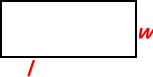Length: Height:
 Plate Both Sides? Yes No

#### Circle: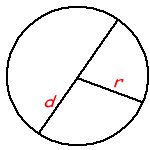Plate Both Sides? Yes No

#### Ellipse: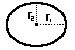r1: r2:
 Plate Both Sides? Yes No

#### Triangle: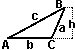Base (b): Height (h):
 Plate Both Sides? Yes No

#### Parallelogram: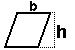Base (b): Height (h):
 Plate Both Sides? Yes No

### Three Dimensional Objects

#### Cube or Brick :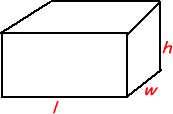Length (l): Width (w): Height (h): Answer:

#### Pyramid:

Calculate the area of all the triangular sides of the pyramid using the triangle tool above. Add the surface area of the base, which may be rectangular, or triangular.

Sphere: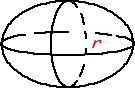#### Egg:#### Cylinder: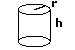#### Tube: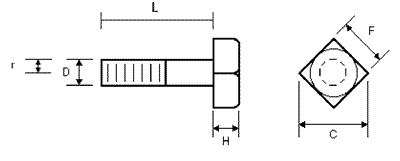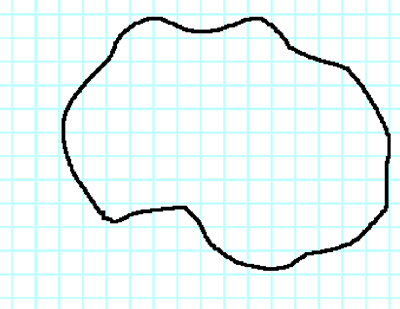To effectively work out a complex surface area, as shown left. Simply place an imaginary grid of 1” squares over the part, and count the whole squares. Then go around the perimeter and add up all the squares that are more than ½ full. Divide the total by 2 and add this to your total of completely filled squares. Another method is to cover the part with one layer of paper or tinfoil. Then remove the tinfoil and cut into smaller pieces so it can be arranged on the floor as close to a rectangle shape as possible. Then simply multiply length x width of the rectangle.